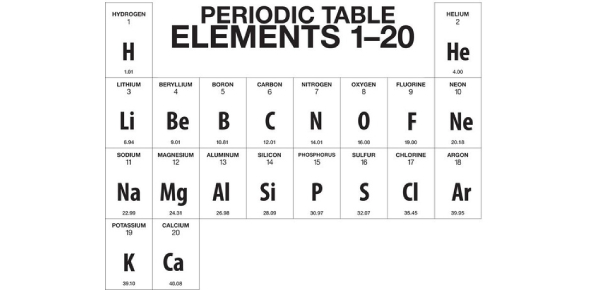# First 20 Elements Of The Periodic Table Quiz

14 Questions | Total Attempts: 61367SettingsHow well do you understand the periodic table and what it involves? Take up the ' first 20 elements of the periodic table quiz below and see if you need to study more about the elements before the next class. The periodic table shows all the chemical elements that exist, their atomic numbers, electron configurations, and chemical properties. Have fun while learning this interesting concept.

• 1.
He stands for...?
• A.

Helium

• B.

Hydrogen

• C.

Hendrox

• 2.
The symbol of Potassium is _________.
• A.

K

• B.

P

• C.

Po

• 3.
What is the symbol of Lithium?
• A.

L

• B.

Li

• C.

Lh

• 4.
Name this element: Be
• 5.
What is the symbol of Argon?
• A.

A

• B.

Ar

• C.

Ag

• 6.
What is the symbol of 'magnesium'?
• A.

M

• B.

Ma

• C.

Mg

• 7.
Which is the symbol of chlorine?
• A.

C

• B.

Ch

• C.

Cl

• 8.
What is the symbol for Hydrogen?
• A.

H

• B.

B

• C.

He

• 9.
What is the symbol for Boron?
• A.

Ho

• B.

B

• C.

Be

• D.

Bo

• 10.
The element 'Be' stands for _____________.
• A.

Oxygen

• B.

Boron

• C.

Beryllium

• D.

Carbon

• 11.
What is the name of this element 'S'?
• A.

Sodium

• B.

Potassium

• C.

Sulphur

• D.

Magnesium

• 12.
What is this element called 'Ca'?
• A.

Calcium

• B.

Potasium

• C.

Carbon

• 13.
The symbol "N" stands for _________.
• A.

Sulphur

• B.

Potassium

• C.

Oxygen

• D.

Nitrogen

• 14.
The symbol "Na" stands for ____________.
• A.

Nitrogen

• B.

Sodium

• C.

Calcium

Related TopicsBack to top